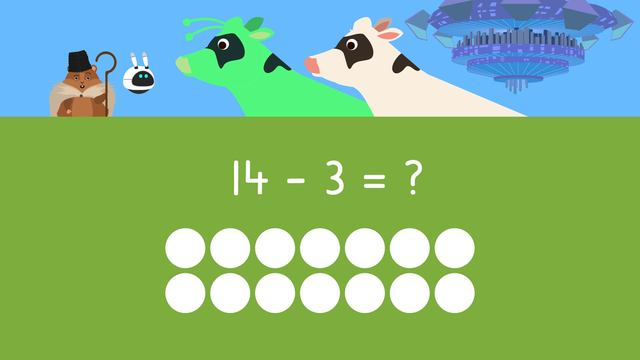# Subtraction Word Problems Up To Twenty

Content Subtraction Word Problems Up To TwentyRating

Ø 4.2 / 5 ratings
The authorsTeam Digital
Subtraction Word Problems Up To Twenty
CCSS.MATH.CONTENT.1.OA.A.1

## Subtraction – Word Problems

What is subtraction? Subtraction word problems are math problems that ask the learner to solve by taking away from a number. In order to solve subtraction problems, you need to do the following:

• The first step is to read or listen to the word problem. As you read or listen, think; what do I need to figure out?’, and highlight the question you need to solve by looking for the question mark.
• The second step is to read or listen to the word problem again and highlight the clue words and numbers. Clue words are words that tell us the important information we need to figure out the answer.
• The third step is to think: Do I add or subtract? How do you know when to subtract in a word problem? There are certain words that mean subtraction in word problems
• The fourth step is to write a number sentence and use drawings to help you solve it!
• The last step is to write down the answer to the subtraction problem.

In the next section we will look at an example of word problems with subtraction.

## Word Problems with Subtraction – Example

This section will explain how to solve subtraction word problems by looking at basic subtraction word problems. Let’s begin: Let’s look at an example of a word problem. The first step is to read the task and to then highlight the clue words. Here we highlight “How many are left?”, because that's what we need to figure out!The second step is to read or listen to the word problem again and highlight the clue words and numbers. Clue words are words that tell us the important information we need to figure out the answer! Here, we highlight, "fourteen cows”, because that's how many cows were there at first. We also highlight, "three cows leave", because that tells us how many left to drink water.The third step is to think: Do I add or subtract? How do you know when to subtract in a word problem? There are certain words that mean subtraction in word problems! In this subtraction word problem, "Three cows leave", which tells us to subtract because they are going away!

The fourth step is to write a number sentence. The number sentence we need to solve is fourteen minus three equals question mark, because there were fourteen cows, and three left to go to the watering hole, so we figure out fourteen minus three to see how many are left!The last step is to solve the number sentence. We can draw circles to help us solve, so we have fourteen circles. Since we are subtracting three, we will cross off three. How many are left?Fourteen minus three equals eleven! So we can answer that eleven cows are left.

## Word Problems with Subtraction – Summary

How do you solve word problems with subtraction? Remember, to find the answer to a word problem using subtraction:

Step # What to do
1 Read or listen to the word problem.
As you read, think; ‘what do I need to figure out?
2 Next, read or listen to it again and
highlight the clue words and numbers.
3 Then, think: ‘Do I add or subtract?’
4 Last, write a number sentence and use drawings